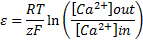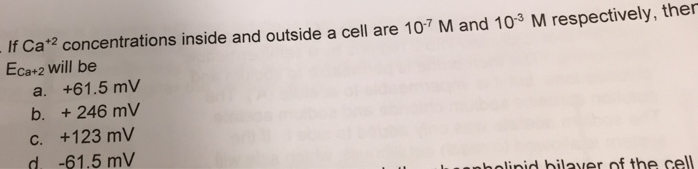# Problem: If Ca+2 concentrations inside and outside a cell are 10-7 and 10-3 M, respectively, then ECa+2 will be: a. +61.5 mVb. +246 mVc. +123mVd. -61.5mV

###### FREE Expert Solution

This would simply make use of the Nernst equation., where

R is the universal gas constant, 8.314 J/mol K

T is the absolute temperature for the system, that is 37ºC for cells  (310 K)

z is the charge of the ion (+2)

F is Faraday's constant (96485 C/mol e)

[Ca+2]out and [Ca+2] in would be the molar concentration of Ca+2 outside and inside of the cell, respectively.###### Problem Details

If Ca+2 concentrations inside and outside a cell are 10-7 and 10-3 M, respectively, then ECa+2 will be:

a. +61.5 mV

b. +246 mV

c. +123mV

d. -61.5mV